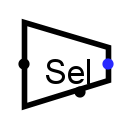#Bit-Wähler

 Bibliothek: Auswahlschaltungen Eingeführt: 2.0.5 Aussehen:## Verhalten

Given an input of several bits, this will divide it into several equal-sized groups (starting from the lowest-order bit) and output the group selected by the select input.

For example, if we have an eight-bit input 01010101, and we are to have a three-bit output, then group 0 will be the lowest-order three bits 101, group 1 will be the next three bits, 010, and group 2 will be the next three bits 001. (Any bits beyond the top are filled in with 0.) The select input will be a two-bit number that selects which of these three groups to output; if the select input is 3, then 000 will be the output.

## Pins (wenn das Bauelement nach Osten zeigt)

West edge (input, bit width matches Data Bits attribute)
Data value from which bits should be selected for the output.
East edge (output, bit width matches Output Bits attribute)
A group of bits from the data value, as selected by the select input.
South edge (input, bit width is quotient of Data Bits and Output Bits, rounded up)
Select input: Determines which of the bit groups should be routed to the output.

## Attribute

When the component is selected or being added, the digits '0' through '9' alter its Output Bits attribute, Alt-0 through Alt-9 alter its Data Bits attribute, and the arrow keys alter its Facing attribute.

Ausrichtung
Die Richtung des Bauelements (Lage des Ausgangs im Vergleich zum Eingang).
Data Bits
The bit width of the component's data input.
Output Bits
The bit width of the component's output.

Keines.

## Verhalten des Textwerkzeugs

Keines.

Zurück zur Bibliotheksreferenz